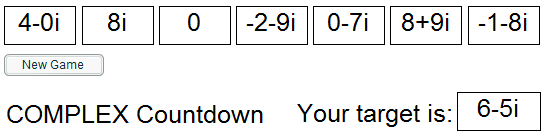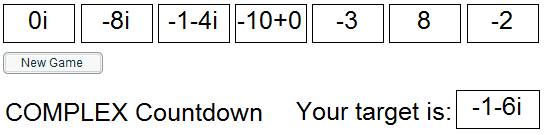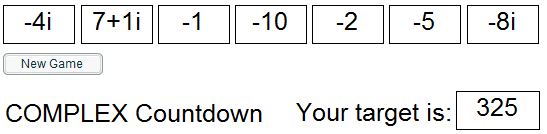#### You may also like### Roots and Coefficients

If xyz = 1 and x+y+z =1/x + 1/y + 1/z show that at least one of these numbers must be 1. Now for the complexity! When are the other numbers real and when are they complex?### Target Six

Show that x = 1 is a solution of the equation x^(3/2) - 8x^(-3/2) = 7 and find all other solutions.### 8 Methods for Three by One

This problem in geometry has been solved in no less than EIGHT ways by a pair of students. How would you solve it? How many of their solutions can you follow? How are they the same or different? Which do you like best?

# Complex Countdown

##### Age 16 to 18 Challenge Level:
Here are a couple of games of complex countdown in action to give you some ideas of how to get started. We use square brackets [ ... ] to show that we are using up one of the original cards.

GAME 1One possible solution here is
[8+9i] + [-2 - 9i]  + [0-7i]  + ([8i]/[4-0i])

GAME 2One possible solution is [-1 - 4i] - [-8i]/(/[-2])

GAME 3Arg[-4i] - (Re[7+1i] x Im[-8i]) + [-1]